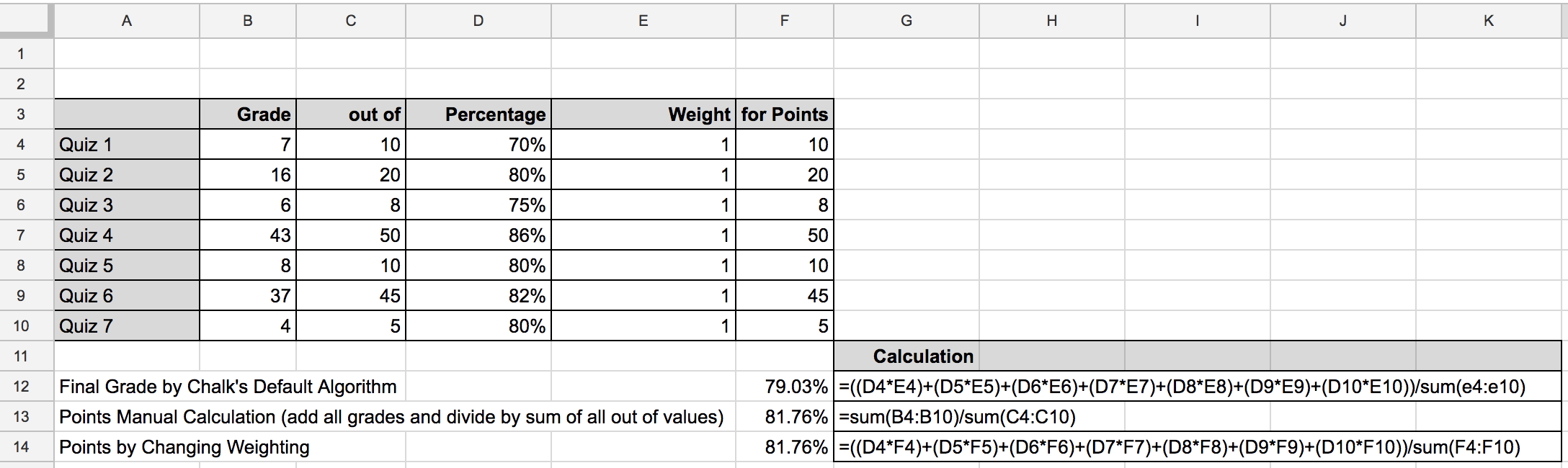# How do I implement a points grading system for a category?

Some teachers and schools want to use a straight points system for calculating grades for their students. There are pros and cons to points based grading and several articles have been written on both sides of the argument (e.g. Point-Based Grading Systems: Benefits and Liabilities).

Grades are calculated in Chalk by using categories and weightings as described in this article but you can implement a points system within a category by manipulating the weighting of each assessment you create.

The following table shows a list of Quizzes delivered to a student and their grades for those quizzes. If each quiz is weighted as 1, the default for Chalk, then the final grade would be calculated by taking the average of all the quizzes to give an average of 79% (rounded).

A points system would sum the Grades for each of the quizzes (121) and divide by the sum of the Out Of for each of the quizzes (148) to give an average of 82% (rounded).

Within Chalk, if you set the weighting of each of the quizzes (here is an article on how to do that) to be the same as the out of value for that assessment, you will effectively be implementing a points system for the final grade. Chalk will multiply the average for the quiz by the weighting for the quiz, adding each of these values for all the quizzes and then divide by the sum of the weightings -- or 82% (rounded).

Here is a table on how the calculations work out:Therefore in order to make one of your categories into a points grading system, you will want to set all of the Weightings (B) to the assessments in this category to the same number as what the Out Of (A) for the assessment is.